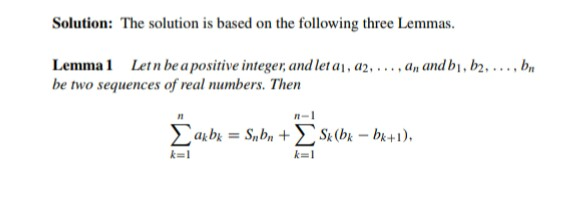# Doubt from trignometry

So we can now conclude that if x \epsilon [0,π] \displaystyle \left|{\sum_{k=1}^n\frac{sin(kx)}{k}}\right| \approx \frac{π-x}{2}<2\sqrt{π}
For high n

How maximum value is {2\sqrt{\pi}} ?? Why u take n high value even question tell any positive value

Bro if x\epsilon R then my greatest integer function will add up

I can insure you that function will never be equal to 2\sqrt{π} @Sneha_2021

Yeah we can conclude that fact but still can't prove it... i thought of pursuing using beta gamma function but did not yeild much results

Bro also Upper bound that function will be very close to y=\frac{π}{2} As n\rightarrow \infty